Thank you for visiting nature.com. You are using a browser version with limited support for CSS. To obtain the best experience, we recommend you use a more up to date browser (or turn off compatibility mode in Internet Explorer). In the meantime, to ensure continued support, we are displaying the site without styles and JavaScript.

# Electric double-layer capacitance between an ionic liquid and few-layer graphene

## Abstract

Ionic-liquid gates have a high carrier density due to their atomically thin electric double layer (EDL) and extremely large geometrical capacitance Cg. However, a high carrier density in graphene has not been achieved even with ionic-liquid gates because the EDL capacitance CEDL between the ionic liquid and graphene involves the series connection of Cg and the quantum capacitance Cq, which is proportional to the density of states. We investigated the variables that determine CEDL at the molecular level by varying the number of graphene layers n and thereby optimising Cq. The CEDL value is governed by Cq at n < 4 and by Cg at n > 4. This transition with n indicates a composite nature for CEDL. Our finding clarifies a universal principle that determines capacitance on a microscopic scale and provides nanotechnological perspectives on charge accumulation and energy storage using an ultimately thin capacitor.

## Introduction

Single-layer graphene (SLG) has two kinds of singularities. One is a topological singularity at K points in non-doped SLG, called the Dirac point. The carrier transport at the Dirac point, with a vanishing density of states D(E), has been intensively studied1,2,3,4,5. The other is the van Hove singularity at M points in highly doped SLG, which has recently attracted significant attention due to predictions of several novel phenomena such as superconductivity6,7, ferromagnetism8 and charge/spin density waves9,10 caused by divergent D(E) and a band structure with high symmetry. We can reach this state by doping SLG with 0.25 electrons or holes per carbon atom (~1 × 1015 /cm2). This high carrier density is expected to be attainable with an ionic-liquid gate because of its thin electronic double layer (EDL)11,12,13,14, i.e. large geometrical capacitance determined by Cg = εILε0/d. Here, εIL, ε0 and d are the relative permittivity of the ionic liquid, vacuum permittivity and thickness of EDL, respectively. However, studies have demonstrated that carrier doping into SLG with ionic liquids is much lower than expected15,16. This is because the potential difference between an ionic liquid and SLG is made smaller than the applied gate voltage Vg by the shift of the Fermi energy EF when doping SLG with an additional charge of Q. This phenomenon can be interpreted in terms of the quantum capacitance Cq, defined as Cq = eQ/EF = e2D(EF)15,17,18, which is connected to Cg in series. Thus, the total capacitance CEDL between the ionic liquid and SLG is understood as follows: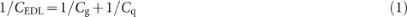The effect of Cq is prominent when Cq Cg, which is characteristic of the combination of SLG with small Cq and an ionic liquid with a large Cg. Thus, even if an ionic liquid has a large Cg, small Cq limits CEDL to make high charge density unattainable. Here, increasing the layer number n might solve this problem, because D(E) increases with n and accordingly Cq increases. To find an optimal n for carrier doping with an ionic liquid is thus quite important for the discovery of novel ordered states in graphene. In addition, the CEDL will increase with n, but the specific surface area (the surface area per unit of mass) of few-layer graphene (FLG) decreases. In a practical viewpoint to realise the superior characteristics in graphene-based supercapacitor19,20, therefore, it is of significance to elucidate the optimal n producing both the large CEDL and specific surface area. In this letter, we examine the capacitance of SLG and FLG while systematically changing n.

## Results

Our results are summarized in Figs. 1a and 1b. The capacitance CEDL between the ionic liquid and FLG was measured as a function of n by two methods, a transport measurement using graphene field effect transistors (FETs) (Fig. 1a) and a capacitance measurement using AC (Fig. 1b). When the gate voltage Vg induces the charge Q(Vg), CEDLDC = Q/Vg and CEDLAC = dQ/dVg are obtained by the transport and capacitance measurements, respectively. These two measurements are complementary to each other and are especially important in the estimation of CEDL, which depends significantly on measurement frequency due to the slow transport of the ions in an ionic liquid. The calculated values of CEDL are also shown in Figs. 1a and 1b and are in good agreement with the experimental data. At n = 1, a small CEDL is observed, as in previous studies15,16. With increasing n, CEDL increases and saturates at n > 4. In the following, we describe the experimental and theoretical details.

First, we evaluated CEDL from transport measurements. Two-terminal graphene FETs were prepared on SiO2 300 nm/highly doped Si substrate. The structure of the device and the optical microscope image are shown in Fig. 2a. In order to obtain a reliable n dependence, we used only uniform graphene layers without any overlaps of different layers, wrinkles or folds. If the device has overlaps of different layers, the exposure of interstices between the neighbouring graphene layers to the ionic liquid might lead to inaccurate n and errors in estimation of surface area. The uniformity of graphene layer was clearly confirmed by the optical contrast of micrographs16,21,22,23,24. Raman spectroscopy mapping and atomic force microscope (AFM) image also evidenced the uniformity of the graphene layer. The number of devices used in this measurement is shown in Table 1; good reproducibility was obtained. The sheet conductivity σ was measured as a function of back gate voltage Vbg applied to the Si substrate. Next, a droplet of ionic liquid was placed on the graphene surface and σ was measured again as a function of the top gate voltage Vtg applied to the ionic liquid with a Pt wire (100 μm in diameter). In Figs. 2b–2d, σs of FLG FETs with n = 1, 4 and 9 are plotted as functions of Vbg and Vtg. Note that the horizontal scales are different for Vbg and Vtg. It was found that σ was modulated with a lower gate voltage for Vtg than Vbg. The conductivity varied with gate voltage in proportion to the capacitance as follows,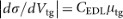or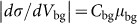, where μ is electric field mobility and the subscript tg (bg) means top (back) gate. Thus, CEDL is given by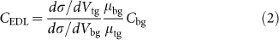Here,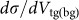is defined as the steepest slope of the experimental data around the charge neutrality point. Assuming μbgtg = 1 and substituting the experimental value of Cbg = 11.5 nF/cm2, CEDL is calculated for each device and plotted in Fig. 1a. A clear n dependence of CEDL is found in Fig. 1a. CEDL is a minimum at n = 1. With increasing n, CEDL shows a maximum at n = 4 and decreases smoothly as n approaches 10. Maximum carrier density was estimated to be 0.003 /C atom (4.7 × 1013 /cm2) at n = 4 and Vtg = 2 V.

In order to prove the validity of CEDL(n) obtained by the transport technique, we directly measured the capacitance using AC. The device structure and the optical microscope image are shown in Fig. 3a. Uniform graphene layers are carefully chosen in this measurement. The graphene layer was covered with photoresist except for a region about 15 μm square, on which a droplet of the ionic liquid was placed. AC voltage was applied across the interface between the ionic liquid and FLG and the capacitance CEDL was evaluated by the out-of-phase components of the detected current. CEDL was measured with an excitation amplitude of 10 mV at a frequency of 500 Hz and with a superimposed DC bias voltage Vtg ranging from −1 to +1 V. The equivalent circuit of our system is shown in Fig. 3a16. Here, it should be noticed that the contact area between the ionic liquid and Pt electrode is much larger than the area between the ionic liquid and FLG. Hence, the impedance of CPt is negligible compared to that of CEDL. This means that Vtg is effectively applied at the interface between the ionic liquid and FLG. Note that Vtg is uniform along this interface, because the impedance of CEDL (~ 108 Ω) is much larger than the resistance of FLG (103 – 104 Ω). The measured impedance is typically Z ~ 106 – 108 i Ω at a frequency of 500 Hz. Therefore, the capacitive component dominates the total impedance, showing that CEDL can be directly determined by the measurement with LCR meter as described in the method section.

In Fig. 3b, CEDL for n = 6 is plotted as a function of Vtg. We found that CEDLs for all the devices significantly depended on Vtg, which corresponds to the ambipolar behaviour in D(E). Here it is important to notice that Vtg in our experiment may be different from the actual voltage applied to the FLG since the potential drop caused by the interface of the Pt wire is not considered. The accurate dc bias in the electrochemical impedance analysis may be defined as the potential difference from the reference electrode using a three-terminal setup15. However, in our devices used in this study, the potential drop at the Pt interface is expected to be so small that applied voltage from the ground is approximately equal to the actual voltage, because the Pt electrode is much wider than the channel region of FLG, as described above. To compare with the transport measurement, the CEDL for each device, obtained at the charge neutrality point, is plotted as a function of n in Fig. 1b. Here the charge neutrality point is defined as the Vtg where CEDL exhibited the lowest value and is located around Vtg = 0 V as shown in Fig. 3b. Since Vtg ~ 0 V, the value of minimum capacitance is obtained without any ambiguities of the bias voltage. Consequently, the discussion on n dependence of capacitance shown in Fig. 1b is appropriate. As shown in Figs. 1a and 1b, a CEDL - n plot is substantially consistent with that from the transport measurement, which supports the validity of our estimation of CEDL from the transport measurement.

## Discussion

Here, we discuss the validity of the CEDL obtained by two-terminal transport measurements. The analysis using equation (2) might oversimplify the estimation of CEDL because the contribution of μbgtg is bypassed. First, we consider the validity of the assumption of μbgtg = 1. This assumption is required because CEDL and μtg cannot be determined independently without the result of Hall effect. In order to investigate the validity of this assumption, we carried out additional transport measurement using the multi-terminal Hall bar devices with n = 1, 2, 7 and 12. (see Supplementary Fig. S2 online). In this method, we can independently determine CEDL and μtg without any assumption. By measuring the Hall coefficient, RH and σ in four-terminal geometry under the gate voltage Vg (Vbg and Vtg), we can evaluate μ (μbg and μtg) and C (Cbg and CEDL) independently by using the following equations25,26.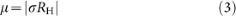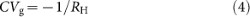These equations are satisfied in the doped region where only one kind of carrier presents. RH was measured as a function of Vtg under perpendicular magnetic field of 6 kOe. The values of CEDL were estimated from the slope of 1/RH - Vtg curve in the range where equation (4) holds. The CEDL - n plot is shown in Fig. S2b and CEDLs are added in the graph in two-terminal measurement (Fig. 1a). This shows a good agreement between the CEDL values obtained from two-terminal transport with assumption of μbgtg = 1 and Hall effect without any assumption. Therefore, this result proves the validity of the assumption of μbgtg ~ 1 in the analysis in two-terminal geometry.

Furthermore, we deduced μbg and μtg separately by substituting both RH and σ in four-terminal geometry into equation (3) and evaluated μbgtg. The result is shown in Fig. S2c. The μbgtg scarcely depends on n and is regarded as a constant around 0.5. The difference, 1 and 0.5, in μbgtg between two analyses described above may be closely associated with the contact resistance. The contact resistance reduces the conductance measured in two-terminal geometry and this effect becomes more significant in highly doped graphene. Accordingly, μtg in two-terminal measurement could be underestimated, because μtg is estimated in more carrier-accumulated region than μbg, while μtg and μbg evaluated from RH and σ measured in four-terminal geometry are not affected by the contact resistance. This may be one of the reasons why the difference in μbgtg takes place between two analyses. It is reasonable that μbgtg using two-terminal mobility enhances from the value (~ 0.5) of μbgtg estimated from Hall bar devices (RH and σ in four-terminal geometry). Thus, μbgtg in two-terminal geometry can be concluded to be close to unity as we assumed in the analysis of equation (2).

Finally, to give physical meaning to the experimental result, we calculated the charge distribution in the FLG that forms the EDL capacitor and obtained CEDL as a function of n. We define UEDL ( = Q2/2CEDL) as the total energy stored in the EDL capacitor where charges +Q and −Q are condensed in the ionic liquid and FLG boundaries, respectively. As shown in Fig. 4a, the charge −Q is distributed over the layers so as to screen penetration of the electric field into the interior. The electric field Ei between the i-th and i+1-th layer becomes smaller than Ei-1 owing to the charge −qi lying on the i-th layer, where i = 1, ..., n is numbered from the ionic liquid side. Then, Cg is obtained by Cg = Q2/2Ug, where Ug is the static field energy due to Ei:where εgr = 5.7 is the relative permittivity of graphite27 and t = 0.34 nm is the distance between graphene layers28. The first term in the right-side expression shows the classical electric field energy accumulated in EDL and the second term indicates that in n-layer graphene. Note that a familiar formula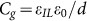is obtained for SLG owing to n = 1. On the other hand, we can relate Cq to the band filling energy Uq29, Cq = Q2/2Uq. Originally Cq was introduced to explain the incomplete shielding of the electric field effect for a two-dimensional electron gas17. We extend this concept to thicker films that can completely screen the electric field by expressing Uq as a summation of the band-filling energy in each layer;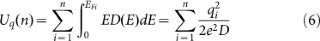where EFi is the Fermi energy of the i-th layer. For simplicity, we used a constant D(E) to deduce the second equality. As mentioned before, Cq = e2D is obtained for SLG. We minimized UEDL ( = Ug + Uq) with respect to each qi of the variational parameters and thereby determined Ug, Uq and UEDL. Using these energies, capacitances were deduced. Cg, Cq and CEDL are plotted as a function of n in Fig. 4b. All the capacitances significantly depend on n: Cq increases and Cg decreases with n and both capacitances saturate to constant values at n 4. This saturation behaviour corresponds to the fact that the electric field is screened by the charge distributed within 3–4 layers29,30 in FLG. Note that the charge distribution over 3–4 layers in FLG significantly reduces Cg because the effective EDL thickness expands from d to ~ d + αt; α is on the order of unity and determined by the effective charge distribution. Therefore, even if an ionic liquid with extremely thin d were used, Cg could not be increased due to the additional EDL thickness of αt (see Supplementary Fig. S4 online). Although layered material is considered to be the most suitable for use with an ionic liquid gate, the effect of decreasing Cg must be taken into account.

In conclusion, we have clarified the microscopic principles determining the capacitance of n-layer graphene by systematic study. The carrier distribution and the band structure in the electrodes of a capacitor define Cg and Cq, respectively, both of which contribute to the total capacitance. This study demonstrates that we may not in principle achieve the high-density carrier accumulation required for EF to reach the van Hove singularity even with ionic liquid gated FLG. This result suggests that we need chemical doping6,31,32 or band control9 to bring the van Hove singularity close to the Fermi energy. Our finding for EDL capacitor may establish a nanotechnological guideline to improve ultrathin capacitors for charge doping, energy storage and electric power supply.

## Methods

FLGs were prepared on a SiO2/highly doped Si substrate by the micromechanical cleavage of Kish graphite (Covalent Materials Co.). The SiO2 was 300 nm thick and its surface was coated with a hydrophobic hexamethyldisilazane (HMDS) layer33 to improve the FET characteristics. The number of graphene layers was distinguished by the contrast of the optical microscope image16,21,22,23,24, atomic force microscopy (AFM) and Raman spectroscopy34,35,36,37 (see Supplementary Fig. S1 online). As described in the main text, we only used uniform graphene layers without any overlaps of different layers, wrinkles and folds in order to obtain a reliable n dependence. FLG devices for two-terminal transport measurement were fabricated by photolithography and the vacuum evaporation of metal (Cr 5 nm/Au 50 nm) and insulator (LiF 30 nm) to passivate the electrodes. FLG devices with a Hall bar structure were prepared using the electron beam lithography and oxygen plasma etching. FLG devices for capacitance measurement were coated with 800 nm thick photoresist (Tokyo Oka, TSMR8900) instead of LiF, followed by photolithography to open a window on the graphene layer. All the measurements were carried out in Ar at room temperature. The transport property was measured with a semiconductor device analyser (Agilent B1500A). We evaluated the conductivity from the slope of IV curves measured at a drain voltage of ±1 mV. Except for the transport measurement using a Hall bar structure, a gel of bmim[PF6] was used as the top gate dielectric (preparation details described elsewhere38). The geometrical capacitance of this ionic liquid was estimated to be 9.7 μF/cm2 by extrapolating the measured capacitance at 20 Hz – 100 kHz to 0 Hz. This value corresponds to d = 0.6 nm, assuming εIL = 7 15. The capacitance measurement was carried out with a precision LCR meter (Agilent E4980A) applying an AC voltage of 10 mV at 500 Hz and superimposed DC voltage Vtg from −1 to 1 V. Only the FLG devices with a Hall bar structure were measured by applying Vtg with an electrolyte LiClO4/PEO. The mixing molar ratio of LiClO4/PEO is 1/20. The geometrical capacitance of this electrolyte is reported to be 15 μF/cm2 39, which is of the same order as that in bmim[PF6].

## References

• Novoselov, K. S. et al. Two-dimensional gas of massless Dirac fermions in graphene. Nature 438, 197–200 (2005).

• Du, X., Skachko, I., Barker, A. & Andrei, E. Y. Approaching ballistic transport in suspended graphene. Nature Nanotech. 3, 491–495 (2008).

• Miao, F. et al. Phase-coherent transport in graphene quantum billiards. Science 317, 1530–1533 (2007).

• Ando, T. Screening effect and impurity scattering in monolayer graphene. J. Phys. Soc. Jpn. 75, 074716 (2006).

• Adam, S., Hwang, E. H., Galitski, V. M. & Das Sarma, S. A self-consistent theory for graphene transport. Proc. Natl. Acad. Sci. USA 104, 18392–18397 (2007).

• McChesney, J. L. et al. Extended van Hove singularity and superconducting instability in doped graphene. Phys. Rev. Lett. 104, 136803 (2010).

• Nandkishore, R., Levitov, L. S. & Chubukov, A. V. Chiral superconductivity from repulsive interactions in doped graphene. Nature Phys. 8, 158–163 (2012).

• Ma, T., Hu, F., Huang, Z. & Lin, H.-Q. Controllability of ferromagnetism in graphene. Appl. Phys. Lett. 97, 112504 (2010).

• Li, G. et al. Observation of Van Hove singularities in twisted graphene layers. Nature Phys. 6, 109–113 (2010).

• Makogon, D., van Gelderen, R., Roldán, R. & Smith, C. M. Spin-density-wave instability in graphene doped near the van Hove singularity. Phys. Rev. B 84, 125404 (2011).

• Ueno, K. et al. Electric-field-induced superconductivity in an insulator. Nature Mater. 7, 855–858 (2008).

• Ye, J. T. et al. Liquid-gated interface superconductivity on an atomically flat film. Nature Mater. 9, 125–128 (2010).

• Bollinger, A. T. et al. Superconductor-insulator transition in La2−xSrxCuO4 at the pair quantum resistance. Nature 472, 458–460 (2011).

• Yamada, Y. et al. Electrically induced ferromagnetism at room temperature in cobalt-doped titanium dioxide. Science 332, 1065–1067 (2011).

• Xia, J., Chen, F., Li, J. & Tao, N. Measurement of the quantum capacitance of graphene. Nature Nanotech. 4, 505–509 (2009).

• Ye, J. et al. Accessing the transport properties of graphene and its multilayers at high carrier density. Proc. Natl. Acad. Sci. USA 108, 13002–13006 (2011).

• Luryi, S. Quantum capacitance devices. Appl. Phys. Lett. 52, 501–503 (1988).

• Das, A. et al. Monitoring dopants by Raman scattering in an electrochemically top-gated graphene transistor. Nature Nanotech. 3, 210–215 (2008).

• Lee, S. H. et al. Three-dimensional self-assembly of graphene oxide platelets into mechanically flexible macroporous carbon films. Angew. Chem. Int. Ed. 49, 10084–10088 (2010).

• Han, T. H. et al. Peptide/graphene hybrid assembly into core/shell nanowires. Adv. Mater. 22, 2060–2064 (2010).

• Blake, P. et al. Making graphene visible. Appl. Phys. Lett. 91, 063124 (2007).

• Ni, Z. H. et al. Graphene thickness determination using reflection and contrast spectroscopy. Nano Lett. 7, 2758–2763 (2007).

• Oostinga, J. B., Heersche, H. B., Liu, X., Morpurgo, A. F. & Vandersypen, L. M. K. Gate-induced insulating state in bilayer graphene devices. Nature Mater. 7, 151–157 (2008).

• Craciun, M. F. et al. Trilayer graphene is a semimetal with a gate-tunable band overlap. Nature Nanotech. 4, 383–388 (2009).

• Novoselov, K. S. et al. Electric field effect in atomically thin carbon films. Science 306, 666–669 (2004).

• Ponomarenko, L. A. et al. Effect of a High-κ Environment on Charge Carrier Mobility in Graphene. Phys. Rev. Lett. 102, 206603 (2009).

• Bechmann, R. Landolt-Börnstein: Zahlenwerte und Funktionen aus Physik Chemie Astronomie Geophysik und Technik, 6 Auflage II Band 6 Teil, Elektrische Eigenshaften I. (Springer-Verlag: Berlin, 1959).

• Castro Neto, A. H., Guinea, F., Peres, N. M. R., Novoselov, K. S. & Geim, A. K. The electronic properties of graphene. Rev. Mod. Phys. 81, 109–162 (2009).

• Kuroda, M. A., Tersoff, J. & Martyna, G. J. Nonlinear screening in multilayer graphene systems. Phys. Rev. Lett. 106, 116804 (2011).

• Miyazaki, H. et al. Inter-layer screening length to electric field in thin graphite film. Appl. Phys. Express 1, 034007 (2008).

• Xue, M. et al. Superconductivity in potassium-doped few-layer graphene. J. Am. Chem. Soc. 134, 6536–6539 (2012).

• Profeta, G., Calandra, M. & Mauri, F. Phonon-mediated superconductivity in graphene by lithium deposition. Nature Phys. 8, 131–134 (2012).

• Lafkioti, M. et al. Graphene on a hydrophobic substrate: doping reduction and hysteresis suppression under ambient conditions. Nano Lett. 10, 1149–1153 (2010).

• Ferrari, A. C. et al. Raman Spectrum of Graphene and Graphene Layers. Phys. Rev. Lett. 97, 187401 (2006).

• Gupta, A., Chen, G., Joshi, P., Tadigadapa, S. & Eklund, P. C. Raman scattering from high-frequency phonons in supported n-graphene layer films. Nano Lett. 6, 2667–2673 (2006).

• Graf, D. et al. Spatially resolved Raman spectroscopy of single- and few-layer graphene. Nano Lett. 7, 238–242 (2007).

• Malard, L. M., Pimenta, M. A., Dresselhaus, G. & Dresselhaus, M. S. Raman spectroscopy in graphene. Phys. Rep. 473, 51–87 (2009).

• Kaji, Y. et al. Characteristics of conjugated hydrocarbon based thin film transistor with ionic liquid gate dielectric. Organic Electron. 12, 2076–2083 (2011).

• Shimotani, H., Asanuma, H., Takeya, J. & Iwasa, Y. Electrolyte-gated charge accumulation in organic single crystals. Appl. Phys. Lett. 89, 203501 (2006).

## Acknowledgements

This work was supported in part by Grants-in-Aid (22244045, 24654105 and 23684028) from MEXT, Japan, by the LEMSUPER project (JST-EU Superconductor Project) and ACT-C project in the Japan Science and Technology Agency (JST) and as a Special Project of Okayama University/MEXT. The transport measurement using Hall bar devices was performed in collaboration with A. Kanda, Y. Ootuka and K. Tsukagoshi. We are grateful to Y. Takabayashi and T. Kambe for comments and suggestions and to M. Mifune for Raman spectroscopy.

## Author information

Authors

### Contributions

H. G. and Y. K. planned the experiments. E. U. prepared graphene devices and carried out the transport and capacitance measurements. All authors analysed and discussed the experimental results.

## Ethics declarations

### Competing interests

The authors declare no competing financial interests.

## Electronic supplementary material

### Supplementary Information

Supplementary information

## Rights and permissions

Reprints and Permissions

Uesugi, E., Goto, H., Eguchi, R. et al. Electric double-layer capacitance between an ionic liquid and few-layer graphene. Sci Rep 3, 1595 (2013). https://doi.org/10.1038/srep01595

• Accepted:

• Published:

• DOI: https://doi.org/10.1038/srep01595

• ### Performance Enhancement and Comparison of Graphene Field Effect Transistor Devices Coated with HMDS Layer

• Manoharan Arun Kumar
• Ramasamy Jayavel
• N. Mohankumar

Silicon (2022)

• ### Green Synthesis of ZnMn2O4 Nanoparticles for Supercapacitor Applications

• J. Uma
• S. Banumathi
• B. Balraj

Journal of Superconductivity and Novel Magnetism (2021)

• ### Interaction of 2D materials with liquids: wettability, electrochemical properties, friction, and emerging directions

• Peter Snapp
• Jin Myung Kim
• SungWoo Nam

NPG Asia Materials (2020)

• ### Ambipolar device simulation based on the drift-diffusion model in ion-gated transition metal dichalcogenide transistors

• Akiko Ueda
• Yijin Zhang
• Yoshihiro Iwasa

npj Computational Materials (2020)

• ### Lithium-ion electrolytic substrates for sub-1V high-performance transition metal dichalcogenide transistors and amplifiers

• Md Hasibul Alam
• Zifan Xu
• Deji Akinwande

Nature Communications (2020)